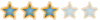# [分享]轉載LISP副程式-抓所有GROUP名稱、查詢GROUP名稱是否存在##[分享]轉載LISP副程式-抓所有GROUP名稱、查詢GROUP名稱是否存在

How do I get the list of named groups being used in Autocad.

`(defun GetGroupList (/ grp grplist)  (setq grp (dictsearch (namedobjdict) "ACAD_GROUP"))  (while (/= (assoc 3 grp) nil)    (setq grpList (cons (cdr (assoc 3 grp)) grpList)     grp     (cdr (member (assoc 3 grp) grp))    )  ) ;_ end of while  (reverse grpList)) ;_ end of defun`

`(defun GroupNameExists (Name)  (null    (vl-catch-all-error-p      (vl-catch-all-apply   'vla-item   (list     (vla-get-groups       (vla-get-activedocument         (vlax-get-acad-object)       )     )     Name   )      )    )  ))`

`(vl-load-com)`RyanGuo##[分享]LISP副程式-取得合乎規範的群組(GROUP)名稱

`;;;新群組名,回傳可行的GROUP NAME或預設群組名稱;;;使用法(SETQ 群組名稱 (NEW_GROUP_NAME 是否可使用已有名稱 預設群組名稱));;;是否可使用已有名稱:1=可以,0=不可以(defun NEW_GROUP_NAME (SN G_NAME / XXX)  (princ "已有GROUP名稱為:\n")  (princ (GETGROUPLIST))  (while (EQ XXX NIL)    (princ      "\n群組名稱最長可以有 31 個字元。"    )    (IF   (EQ SN 0)      (princ   "群組名稱不可重複。"      )    )    (SETQ XXX (getstring      (strcat   "\n請輸入新群組名稱(或使用預設名稱: <"         G_NAME         "> ):"      )         )    )               ;取得新群組名稱    (cond      ((EQ XXX "")       (SETQ XXX G_NAME)      ) ;_cond 第1 END      ((GroupNameExists XXX)       (progn    (IF (EQ SN 1)      (progn        (initget "Y N")        (IF          (EQ       (getkword         "名稱與已有GROUP名稱相同,是否要使用這個名稱[Y/N]<N>:"       )       "Y"          )      (SETQ G_NAME XXX)      (SETQ XXX NIL)        ) ;_IF結束      );_progn結束      (SETQ XXX NIL)    ) ;_IF結束       ) ;_progn結束      ) ;_cond 第2 END      ((> (strlen XXX) 32)       (SETQ XXX NIL)      ) ;_cond 第3 END      (T       (SETQ G_NAME XXX)      ) ;_cond 第4 END    ) ;_cond結束  ) ;_while結束) ;_副程式NEW_GROUP_NAME結束`

[分享]轉載LISP副程式-抓所有GROUP名稱、查詢GROUP名稱是否存在

`(defun C:TE (/ GNN)  (SETQ GNN (NEW_GROUP_NAME 1 "預設群組名稱"))  (princ GNN))`RyanGuo##[分享]LISP副程式-取得升/降序連續整數LIST,給FOREACH用

`;;回傳升/降序連續整數;;(LIST4FOREACH n mot);;mot=[nil,"R","Z","RZ"]=[沒反轉沒零,反轉沒零,不反轉有零,反轉有零](defun LIST4FOREACH (n mot / lis)  (IF (/= (TYPE n) 'INT)    (progn      (PRIN1 "副程式LIST4FOREACH發生錯誤,n不等於整數.")      (EXIT)    )    (repeat (IF   (OR (= mot "Z") (= mot "RZ"))         (1+ n)         n       )      (SETQ   lis (cons n lis)   n   (1- n)      )    )  ) ;_IF  (IF (OR (= mot "R") (= mot "RZ"))    (reverse lis)    lis  ) ;_IF) ;_defun LIST4FOREACH`RyanGuo##[分享]LISP副程式-list排序副程式

`;;list排序副程式,需搭配code:str使用;;回傳list為第1個元素開始排序到第n個,排序方式為升序;;支援文字首字code排序;;(sort:list list:A n)(defun sort:list (list:A n)  (repeat (IF (/= (TYPE n) 'INT)       ;;n不為int時,自動抓取list:A的第一個元素數量       (SETQ n (length (car list:A)))       n     )    (SETQ n    (1- n)     list:A (vl-sort list:A           (function             (lambda (E1 E2)               (< (code:str (nth n E1)) (code:str (nth n E2)))             ) ;_lambda           )       )    )  )) ;_sort:list`

`;;回傳字串首字的code;;若為int或real則回傳原本數值,其他類型回傳0(defun code:str   (in)  (COND    ((= (TYPE in) 'STR)     (ascii in)    )    ((OR (= (TYPE in) 'INT) (= (TYPE in) 'REAL))     in    )    (T 0)  )) ;_code:str`

`(SETQ list:A       (vl-sort   list:A      (function        (lambda (E1 E2)          (< (code:str (nth 1 E1)) (code:str (nth 1 E2)))        ) ;_lambda      )       ))`RyanGuo##[分享]LISP副程式-位元值控制副程式

`               ;位元值判斷,依位元值的T或NIL回傳T或NIL;;(bw_ton 被判斷值 判斷值)(defun bw_ton (int1 bit_code)  (/= (logAnd int1 bit_code) 0)) ;_bw_ton`

`               ;位元值變動,回傳變動後數值;;(bw_ex 被判斷值 判斷值)(defun bw_ex (int1 bit_code)  (IF (/= (logAnd int1 bit_code) 0)    (- int1 bit_code)    (+ int1 bit_code)  )) ;_bw_ex`RyanGuo